“机器学习是近20多年兴起的一门多领域交叉学科，涉及概率论、统计学、逼近论、凸分析、算法复杂度理论等多门学科。机器学习理论主要是设计和分析一些让 可以自动“ 学习”的算法。机器学习算法是一类从数据中自动分析获得规律，并利用规律对未知数据进行预测的算法。因为学习算法中涉及了大量的统计学理论，机器学习与统计推断学联系尤为密切，也被称为统计学习理论。算法设计方面，机器学习理论关注可以实现的，行之有效的学习算法。很多 推论问题属于 无程序可循难度，所以部分的机器学习研究是开发容易处理的近似算法。” ——中文维基百科0+0+0+0+0+0+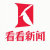0+0+0+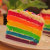0+0+0+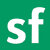【机器学习在马蜂窝酒店聚合中的应用初探】分享自 @SegmentFault，传送门：http://t.cn/A6vou2Ep0+0+0+0+0+0+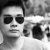0+0+0+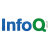#小Q分享# 《AI 风投的下一个拐点是进还是退？》：0+0+0+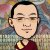0+0+0+0+0+0+0+0+0+0+0+0+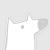0+0+0+0+0+0+0+0+0+
Top